# Forestry: Population Growth and Differential Equations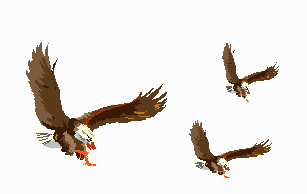The growth of many natural populations depends on the population’s density. When the population is low, its growth is high. As the population grows, there is more competition for food, predators become more abundant, and its growth slows. A quantity called the carrying capacity is the maximum density that a particular habitat can sustain. For sparse populations, growth is often proportional to the density, and for populations close to the carrying capacity, growth is roughly proportional to the difference between the carrying capacity and the population.

If C is the carrying capacity, and k is a constant of proportionality representing the population’s growth rate when the population density is very low, one way to summarize the above is the equation,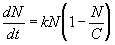(equation 1)

where N is the population density at time t, and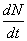is the rate of change in the population density. Observe that when N is small, then approximately,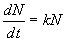because N is much smaller than C. Hence, there is exponential growth for small N. However, as N increases toward C, the growth rate diminishes and when N is close to C, we have, approximately,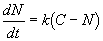which approaches zero as N approaches C. Thus equation (1) satisfies the description given above. Now we need to solve this differential equation for the population density (N) in terms of the time (t).

## Solution:

We can rearrange the equation to isolate t: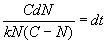Integrating both sides,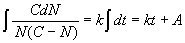where A is a constant. Using algebra, we can rearrange the terms on the left side of the equation as follows: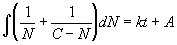The left side can be integrated using the fact that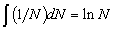resulting in the equation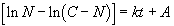3) Finally, we solve for N to obtain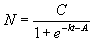(equation 2)

## Worked example:

Suppose for bald eagles in a certain area, the carrying capacity of the habitat is estimated to be 14.2 eagles/km2., and the rate of growth for a sparse population is k = 40% per year. A survey done in 1998 shows that the population density was 3.1 eagles/km2. What do you estimate the population be in each year between 1995 and 2010 if environmental conditions remain the same, i.e. that the carrying capacity, C, and the growth rate, k, remain unchanged?

### Step 1: Compute the unknown constant, A, from initial conditions.

We will measure the time in years, referring to 1998 as year t = 0. When t = 0, equation (2) becomes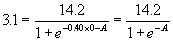Standard algebra yields A = -1.2755. Thus the population density and time in years are related by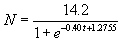(equation 3)

### Step 2: Compute N for values of t of interest and display the results.

For 1995, t = -3 and for 2010, t = 12. Substitute each integer between -3 and 12 into the above equation and compute N. If the information is graphed, with the year, t + 1998, on the x-axis and the population density, N, on the y-axis, figure 1 is the result.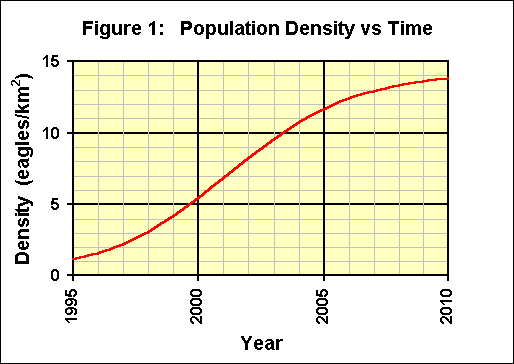When interpreting such results, remember some of the basic assumptions:

• The carrying capacity, C, does not vary over the period of the analysis.
• The growth rate, k, does not vary over the period of the analysis.
• The form of the growth is adequately described by the equation

### Step 3: Discussion of the results.

Note that during the first few years when the density is much less than the carrying capacity, the growth seems to be exponential. As the density approaches the carrying capacity, the growth declines and the density will not exceed the carrying capacity.Written by G. John Smith, June 11, 1998